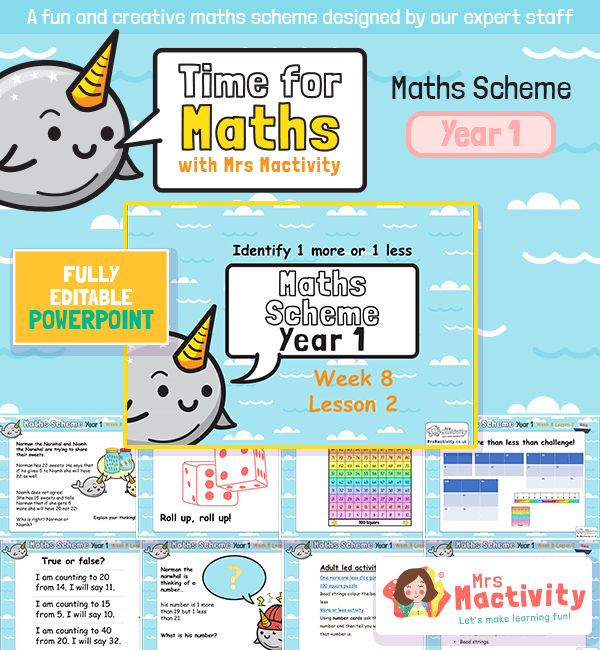# One More One Less Maths PowerPointNew! Time for maths – the new maths scheme of work by Mrs Mactivity! Our maths scheme of work consists of daily PowerPoints with accompanying teacher notes and resources that can be easily used by teachers who want to save time and delivery interesting maths lessons that help children make progress. Our maths scheme centres around a maths mastery approach with plenty of opportunities to develop fluency and confidence in maths.

This particular lesson – One More One Less Maths PowerPoint Maths Scheme Year 1 Week 8 Lesson 2 – covers the following National Curriculum objectives:

• given a number, identify one more and one less.
• count to and across 100, forwards and backwards, beginning with 0 or 1, or from any given number
• count, read and write numbers to 100 in numerals; count in multiples of twos, fives and tens
• add and subtract one-digit and two-digit numbers to 20, including zero
• solve one-step problems that involve addition and subtraction,

Explore the rest of our maths scheme of work.

Free

#### Recently Viewed